## 1. Transformer

Transformer详解:

### 1.1. Encoder-Decoder

Transformer结构可以表示为Encoder和Decoder两个部分: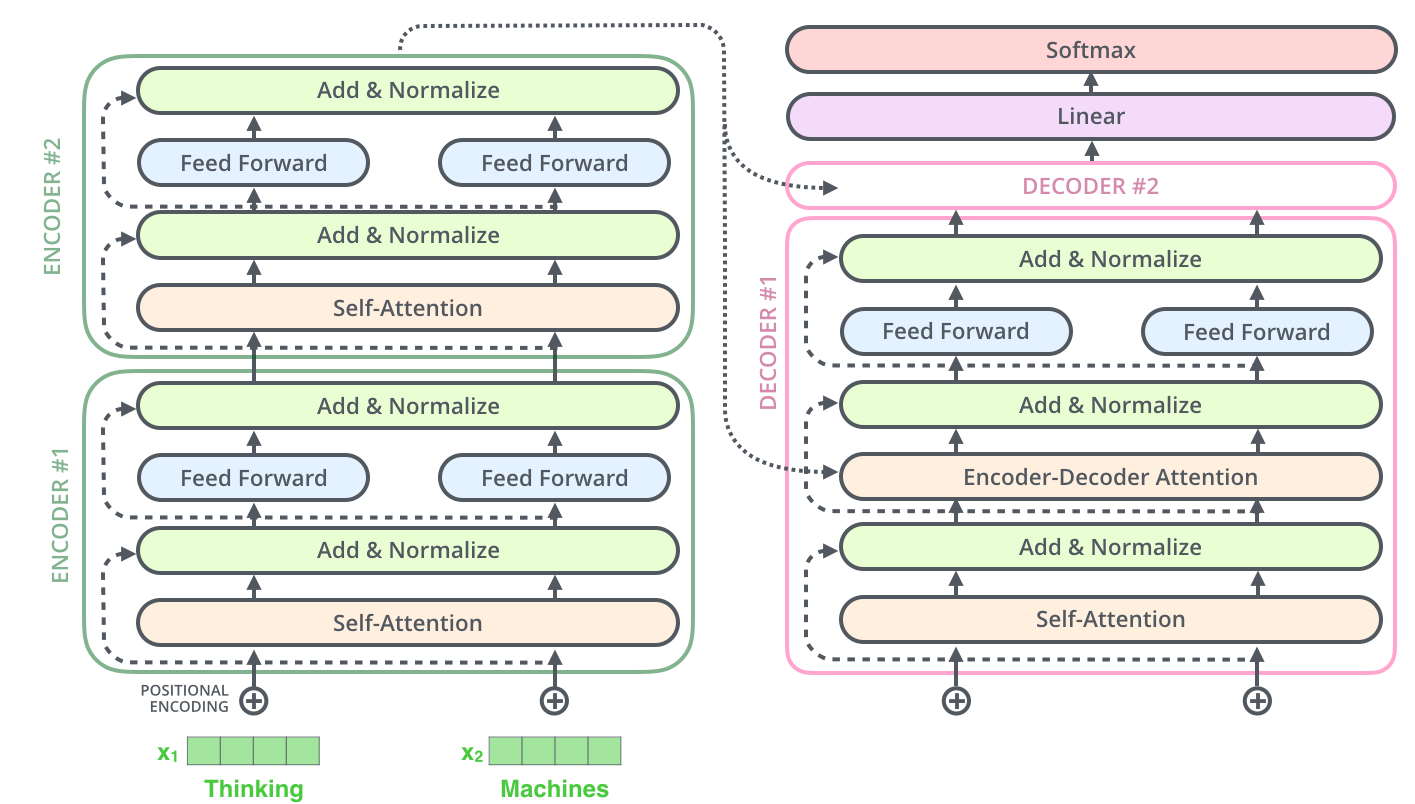Encoder 和 Decoder 主要由 Self-AttentionFeed-Forward Network 两个组件构成，Self-Attention 由 Scaled Dot-Product Attention 和 Multi-Head Attention 两个组件构成.

Scaled Dot-Product Attention公式：

$$\text{Attention} (Q, K, V) = \text{softmax} (\frac{QK^T}{\sqrt{d_k}}) V$$

$$\text{MultiHead}(Q, K, V) = \text{Concat} (\text{head}_1, ..., \text{head}_{\text{h}} W^Q)$$

Feed-Forward Network公式：

$$\text{FFN}(x) = \text{max}(0, xW_1 + b_1) W_2 + b_2$$

### 1.2. Positional Encoding$$PE_{(pos, 2i)} = \text{sin} (\frac{pos}{10000^{\frac{2i}{d_{model}}}})$$

$$PE_{(pos, 2i+1)} = \text{cos} (\frac{pos}{10000^{\frac{2i}{d_{model}}}})$$

### 1.3. Self-Attention

#### 1.3.1. Scaled Dot-Product Attention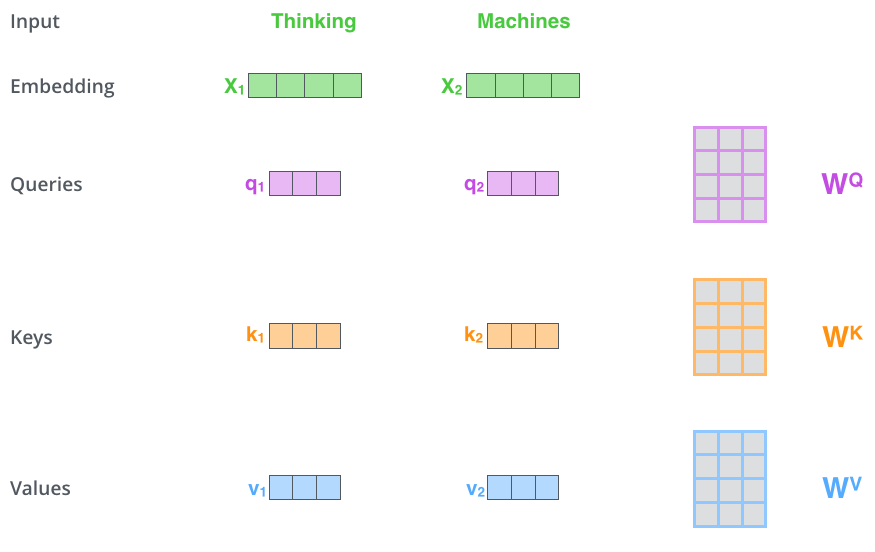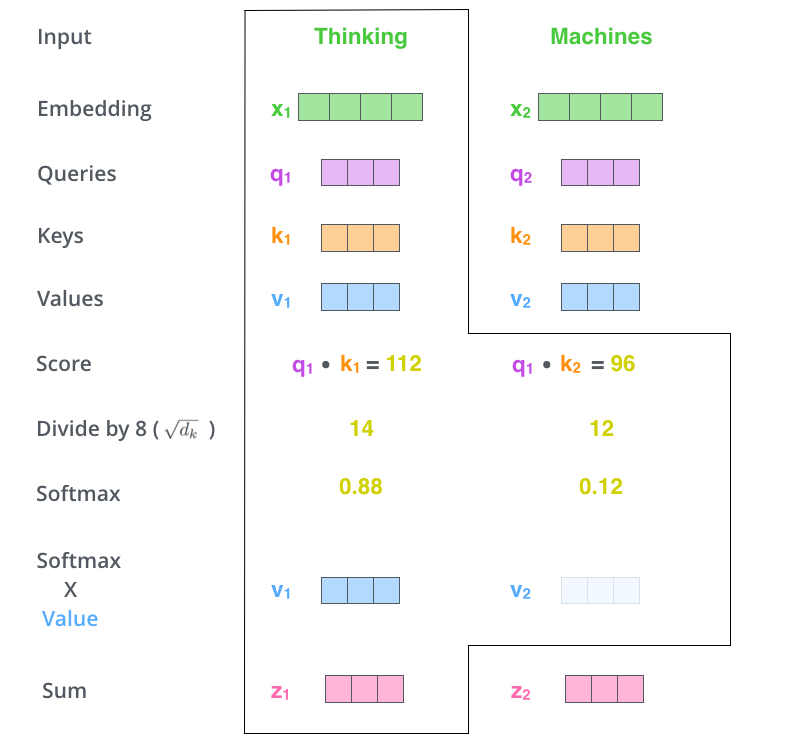- 每个输入单词转化为嵌入向量；

 - 根据嵌入向量得到 q, k, v 三个向量；

 - 通过 q, k 向量计算 score: $score = q \cdot k$；

 - 对 score 进行归一化，即除以 $\sqrt{d_k}$；

 - 通过 softmax 激活函数计算 score；

 - softmax 点乘 Value 值 v，得到每个输入向量的评分 v；

 - 所有输入向量的评分 v 之和为 z: $z \sum v$.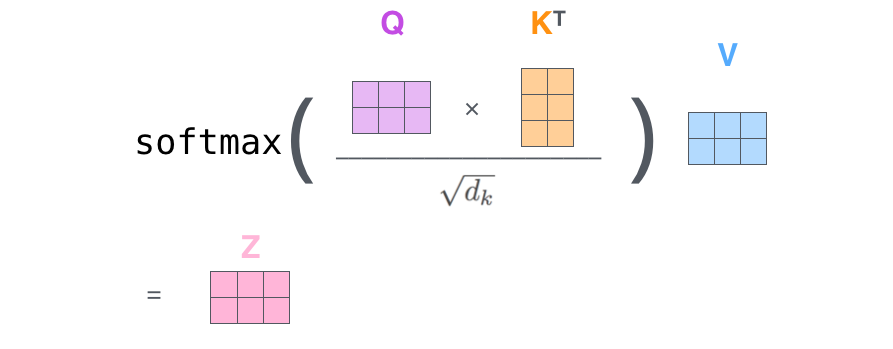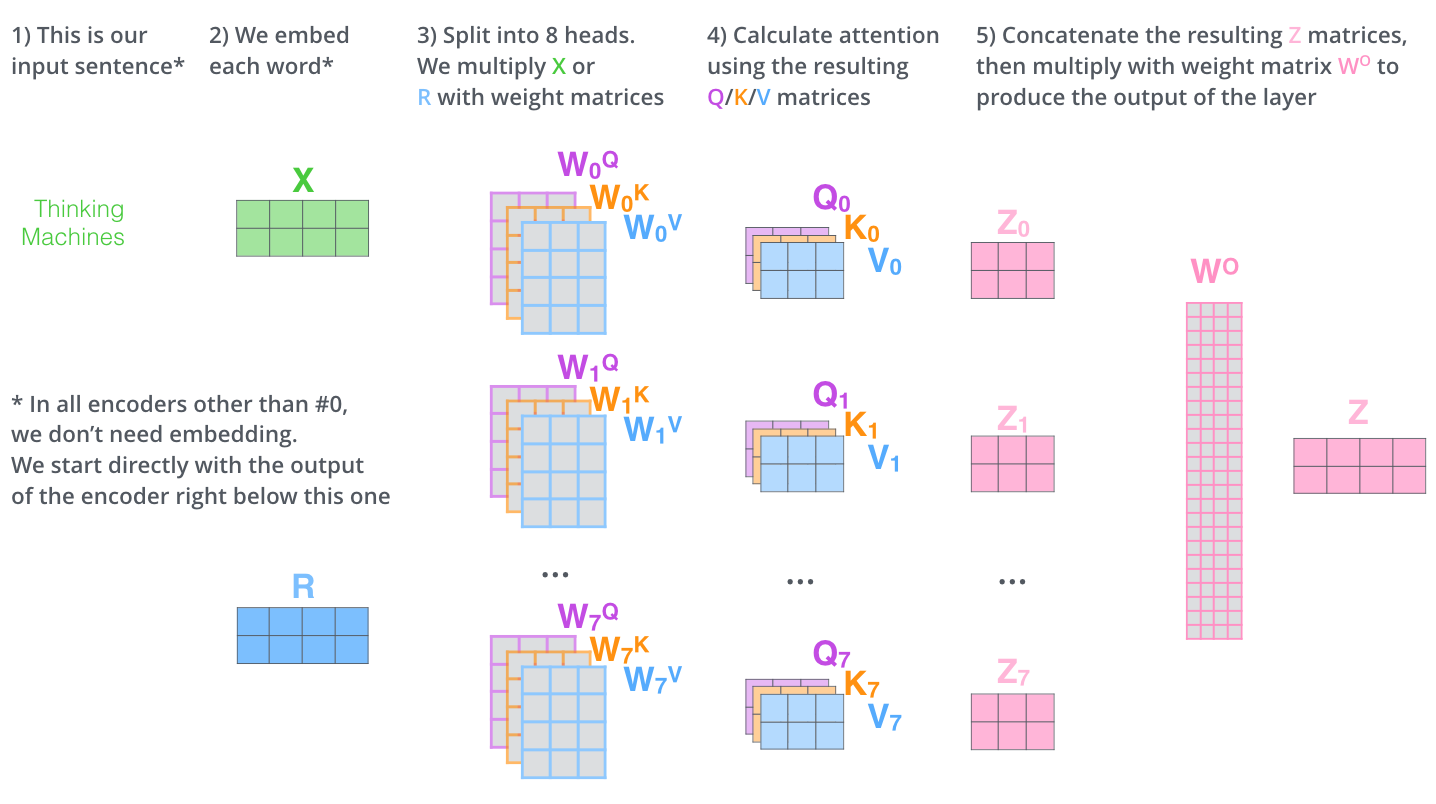- 将数据 $X$ 分别输入到 8 个不同的 Scaled Dot-Product Attention 中，得到8个加权后的特征矩阵 $Z_i, i \in \lbrace 1, 2, ..., 8 \rbrace$；

 - 将 8 个 $Z_i$ 按列拼成一个大的特征矩阵；

 - 特征矩阵经过一层全连接得到输出 $Z$.

Scaled Dot-Product Attention 和 Multi-Head Attention 都加入了 short-cut 机制.

## 2. Vision Transformer(ViT)

ViT 将 Transformer 巧妙的应用于图像分类任务，更少计算量下性能跟SOTA相当.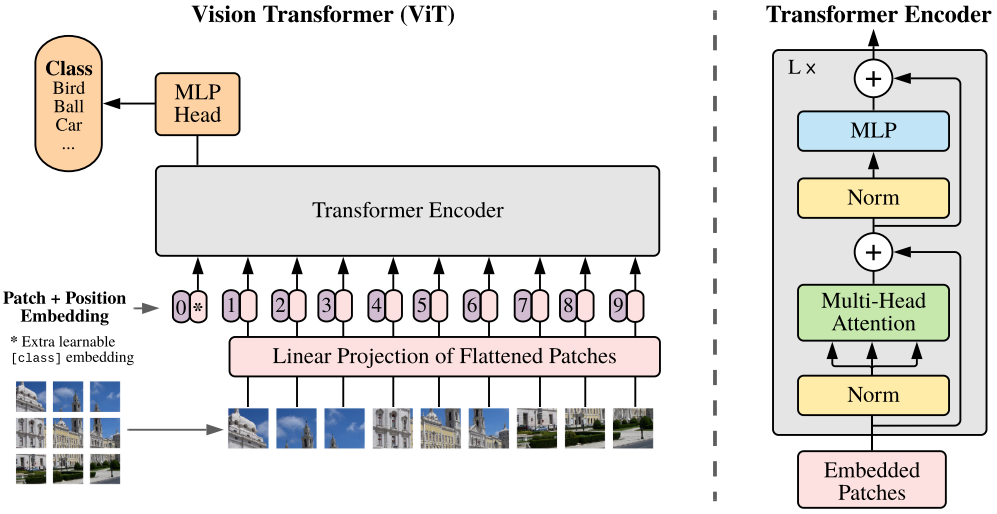Vision Transformer(ViT) 将输入图片拆分成16x16个patches，每个 patch 做一次线性变换降维同时嵌入位置信息，然后送入 Transformer，避免了像素级 attention 的运算.

$$\pmb{z}_0 = [\pmb{x}_{\text{class}}; \pmb{x}_{\text{p}}^{1}\pmb{E}; \pmb{x}_{\text{p}}^{2}\pmb{E}; \cdots \pmb{x}_{\text{p}}^{N}\pmb{E} ] + \pmb{E}_{\text{pos}}$$

$\pmb{E} \in R ^{P^2 \cdot C} \times D$；

$\pmb{E} \in R ^{(N+1) \times D}$.

$$\pmb{z} _l^{'} = \text{MSA} (\text{LN}(\pmb{z}_{l-1})) + \pmb{z}_{l -1}$$

$$\pmb{z} _l = \text{MLP} (\text{LN}(\pmb{z} _l^{'})) + \pmb{z} _l^{'}$$

$$\pmb{y} = \text{LN}(\pmb{z} _l^{0})$$

$(H, W)$ 为原图像分辨率，$(P, P)$ 为每个图像 patch 的分辨率，$N = \frac{HW}{P^2}$ 为 Transformer 输入序列的长度.

ViT 舍弃了CNN的归纳偏好问题，更加有利于在超大规模数据上学习知识，即大规模训练优归纳偏好，在众多图像分类任务上直逼SOTA.

## 3. DETR

DETR 使用 set loss function 作为监督信号来进行端到端训练，然后同时预测所有目标，其中 set loss function 使用 bipartite matching 算法将 pred 目标和 gt 目标匹配起来. 直接将目标检测任务看成set prediction 问题，使训练过程变的简洁，并且避免了anchor、NMS等复杂处理.

DETR主要有两个部分：architecture 和 set prediction loss.

### 3.1. Architecture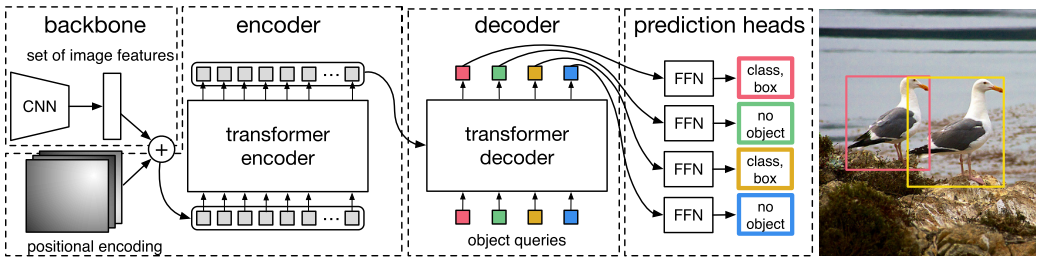- DETR 先用 CNN 将输入图像 embedding 成一个二维表征;

 - 然后将二维表征转换成一维表征并结合 positional encoding 一起送入 encode;

 - decoder 将少量固定数量的已学习的 object queries(可以理解为 positional embeddings)和 encoder 的输出作为输入.

 - 最后将 decoder 得到的每个 output embdding 传递到一个共享的前馈网络(FFN)，该网络可以预测一个检测结果(包括类和边框)或着“没有目标”的类.

#### 3.1.1. Transformer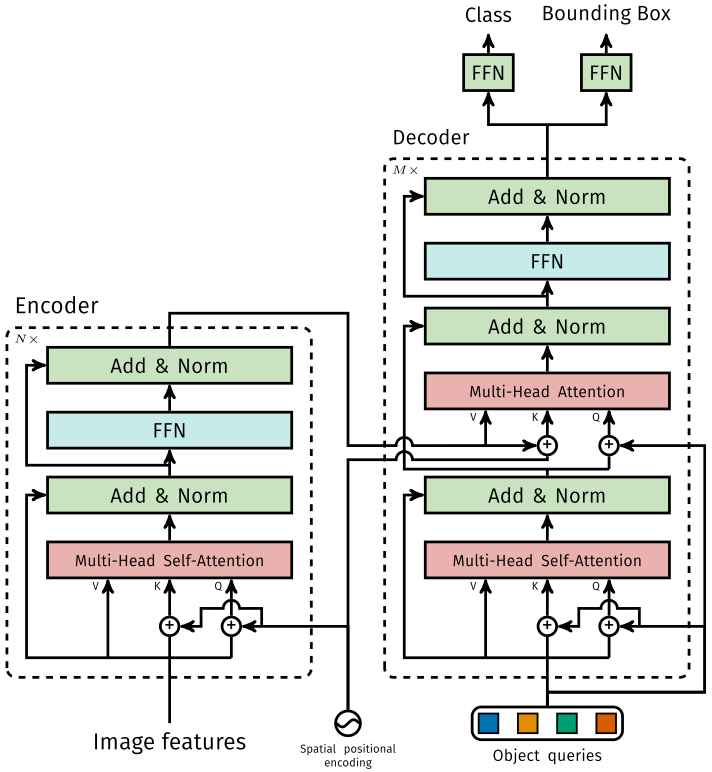- Encoder

 - Decoder

 - FFN

FFN 由 3 层 perceptron 和一层 linear projection 组成. FFN 预测出 box 的归一化中心坐标、长、宽和class.

DETR 预测的是固定数量的 $N$ 个box的集合，并且 $N$ 通常比实际目标数要大的多，所以使用一个额外的空类来表示预测得到的box不存在目标.

### 3.2. Set prediction loss

DETR 模型训练的主要困难是如何根据 gt 衡量预测结果(类别、位置、数量).

DETR 提出的 loss 函数可以产生 pred 和 gt 的最优双边匹配(确定pred和gt的一对一关系)，然后优化loss.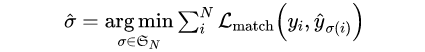hungarian loss公式如下：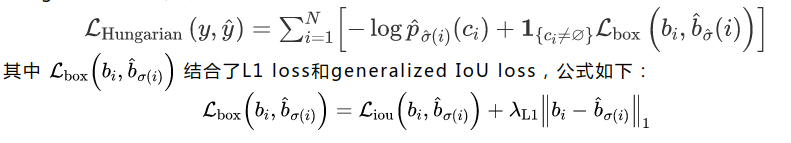ViT和DETR两篇文章的实验和可视化分析很有启发性，感兴趣的可以仔细看看~~

## 4. Deformable DETR

Deformable DETR 将 DETR 中的 attention 替换成 Deformable Attention，使DETR范式的检测器更加高效，收敛速度加快10倍.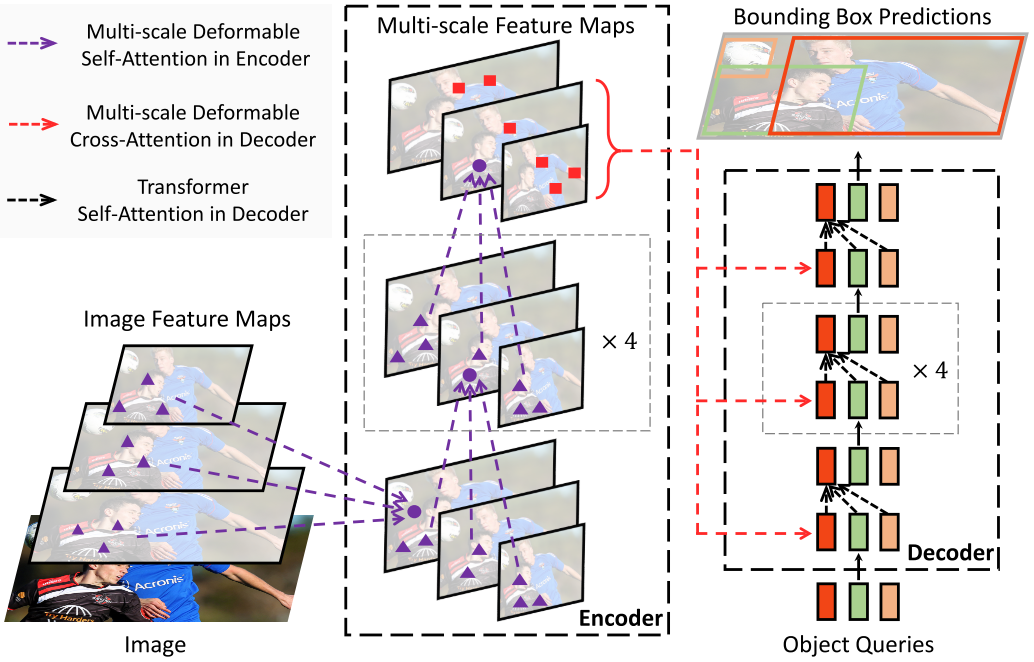Deformable DETR 提出的 Deformable Attention 可以缓解 DETR 的收敛速度慢和复杂度高的问题. 同时结合了 deformable convolution 的稀疏空间采样能力和transformer的关系建模能力.

Deformable Attention 可以考虑小的采样位置集作为一个pre-filter突出所有feature map的关键特征，并且可以自然地扩展到融合多尺度特征，并且Multi-scale Deformable Attention本身就可以在多尺度特征图之间进行交换信息，不需要FPN操作.

### 4.1. Deformable Attention Module

$$\text{MultiHeadAttn}(\mathcal{z}_1, x) = \sum_{m=1}^{M} W_m [\sum_{k \in \Omega_k} A_{mqk} \cdot W_{m}^{'} x_k]$$

$$\text{Attn}(\mathcal{z}_q, x) = \sum_{m=1}^M W_m [\sum_{k=1}^K A_{mqk} \cdot W_{m}^{'} x]$$

DETR 主要有两个问题：需要更多的训练时间来收敛对小目标的检测性能相对较差. 本质上是因为 Transfomer 的 Multi-Head Attention 会对输入图片的所有空间位置进行计算.

$$\text{DeformAttn}(\mathcal{z}_q, p_q, x) = \sum_{m=1}^{M} W_m [\sum_{k=1}^{K} A_{mqk} \cdot W_{m}^{'} x (p_q + \Delta p_{mqk})]$$

$\Delta p_{mqk}, A_{mqk}$ 分别表示第 $k$ 个采样点在第 $m$ 个 attention head 的采样偏移量和注意力权重. 注意力权重 $A_{mqk}$ 在 [0, 1] 范围内，且归一化 $\sum_{k=1}^K A_{mqk} = 1$.

$\Delta p_{mqk} \in R ^2$ 表示为无约束范围的二维实数.

### 4.2.2. Multi-scale Deformable Attention Module

Deformable Attention可以很自然地扩展到多尺度的feature maps.

$\lbrace x^l \rbrace$ 表示为输入的多尺度feature maps，$x^l \in R ^{C \times H_l \times W_l}$.

$\hat{p}_q \in [0, 1]^2$ 表示为每个query元素 $q$ 的参考点 $p$ 的归一化坐标.

Deformable DETR 的 Multi-scale Deformable Attention公式表示为：

$$\text{MSDeformAttn}(z_q, \hat{p}_q, \lbrace x^l \rbrace _{l=1}^L) = \sum_{m=1}^M W_m [\sum_{l=1}^L \sum_{k=1}^K A_{mlqk} \cdot W_m^{'} x^l (\phi_l(\hat{p}_q) + \Delta p_{mlqk})]$$

$\Delta p_{mlqk}, A_{mlqk}$ 分别表示第 $k$ 个采样点在第 $l$ 特征层的第 $m$ 个 attention head 的采样偏移量和注意力权重. 注意力权重 $A_{mlqk}$ 在 [0, 1] 范围内，且归一化 $\sum_{k=1}^K A_{mlqk} = 1$.

### 4.2.3. Deformable Transformer Encoder

Encoder 的输入和输出都是具有相同分辨率的多尺度feature maps.

Encoder 从 ResNet 的 C3-C6 中抽取多尺度 feature maps $\lbrace x^l \rbrace _{l=1}^L (L=4)$，(C6 由 C5 进行 3x3 stride 2 卷积得到).

### 4.2.4. Deformable Transformer Decoder

Decoder 中有 cross-attention 和 self-attention 两种注意力. 这两种注意力的 query 元素都是 object queries.

## 5. 复杂度分析

### 5.1. Convolution 复杂度

 - 为了保证输入和输出在第一个维度都相同，故需要对输入进行padding操作，因为这里 kernel size 为 $k$ (实际 kernel 的形状为 $k \times k \times d$).

 - 大小为 $k \times k$ 的卷积核一次运算复杂度为 $O(k^2 d)$，一共做了 $H \times W$ 次，姑复杂度为 $O(HW k^2 d)$.

 - 为了确保第三个维度相等，故需要 $d$ 个卷积核，所以卷积操作的时间复杂度为 $O(HWK^2 d^2)$.

### 5.2. Self-Attention 复杂度

$$\text{Attention} (Q, K, V) = \text{softmax} (\frac{QK^T}{\sqrt{d_k}}) V$$

 - $Q, K, V$ 的计算复杂度为 $n \times d$.

 - 相似度计算 $QK^T$: $n \times d$ 与 $d \times n$ 运算，得到 $n \times n$ 矩阵，复杂度为 $O(n^2 d)$.

 - softmax 计算：对每行做 softmax，复杂度为 $O(n)$，则 $n$ 行的复杂度为 $O(n^2)$.

 - 加权和：$n \times n$ 与 $n \times d$ 运算，得到 $n \times d$ 矩阵，复杂度为 $O(n^2 d)$.

 - 最后，Self-Attention 的时间复杂度为 $O(n^2 d)$.

### 5.3. Transformer 复杂度

Self-Attention 的时间复杂度为 $O(n^2 d)$.

### 5.4. ViT

$N = H \times W / P^2$.

Self-Attention 的复杂度为 $O(H^2 W^2 d/P^4)$.

### 5.5. DETR

$n = H \times W$

Self-Attention 的复杂度为 $O(H^2 W^2 d)$.

### 5.6. Deformable DETR

Self-Attention的复杂度为 $O(HWd^2)$.

## 6. 几个问题

### 6.1. Q，K，V 如何理解？为什么不使用相同的 Q 和 K?

 - 从点乘的物理意义上讲，两个向量的点乘表示两个向量的相似度.

 - $Q, K, V$ 的物理意义是一样的，都表示同一个句子中不同 token 组成的矩阵. 矩阵的每一行，是表示一个 token 的 word embedding 向量. 假设一个句子“Hello, how are you?” 长度是6，embedding 维度是300，那么 $Q, K, V$ 都是 (6, 300) 的矩阵.

https://medium.com/dissecting-bert/dissecting-bert-part-1-d3c3d495cdb3

### 6.4. 归纳偏好是什么？### 6.5. 二分图匹配? 匈牙利算法？

https://liam.page/2016/04/03/Hungarian-algorithm-in-the-maximum-matching-problem-of-bigraph/

https://zhuanlan.zhihu.com/p/62981901

### 6.6. BETR 的positional embedding、object queries和slot三者之间有何关系？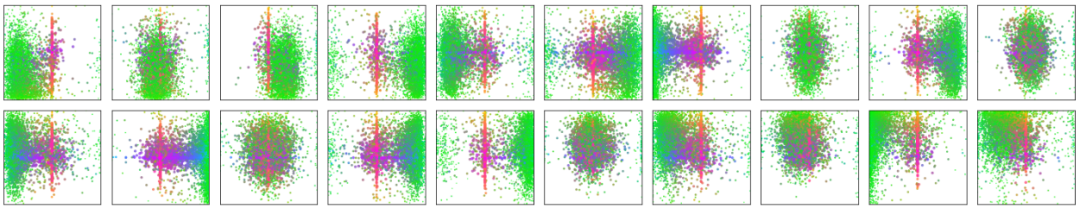DETR可视化 decoder 预测得到的 20 个slot. 可以观察到每个slot学习到了特定区域的尺度大小. Object queries 从这个角度看，其实有点像 Faster-RCNN 等目标检测器的anchor，结合 encoder 的positional embedding 信息让每个 slot 往学习到的特定区域去寻找目标.

### 6.7. Transformer相比于CNN的优缺点?

Transformer关注全局信息，能建模更加长距离的依赖关系，而CNN关注局部信息，全局信息的捕捉能力弱.

Transformer避免了CNN中存在的归纳偏好问题.

Transformer复杂度比CNN高，但是ViT和Deformable DETR给出了一些解决方法来降低Transformer的复杂度.

## 7. 总结

Transformer给图像分类和目标检测方向带来了巨大革新，分割、跟踪、视频等方向也不远了吧

NLP和CV的关系变的越来越有趣了，虽然争议很大，但是试想一下，NLP和CV两个领域能用一种范式来表达，该有多可怕，未来图像和文字是不是可以随心所欲的转来转去？可感知可推理的强人工智能是不是不远了？(想想就好)

## Reference

 - Attention Is All You Need

 - An Image is Worth 16*16 Words: Transformers for Image Recognition at Scale

 - End-to-End Object Detection with Transformers

 - Deformable DETR: Deformable Transformers for End-to-End Object Detection

Last modification：June 16th, 2021 at 11:04 am